# New features in qgraph 1.5

Written by Sacha Epskamp.

While the developmental version is routinely updated, I update the stable qgraph releases on CRAN less often. The last major update (version 1.4) was 1.5 years ago. After several minor updates since, I have now completed work on a new larger version of the package, version 1.5, which is now available on CRAN. The full list of changes can be read in the NEWS file. In this blog post, I will describe some of the new functionality.

# New conservative GGM estimation algorithms

Recently, there has been some debate on the specificity of EBICglasso in exploratory estimation of Gaussian graphical models (GGM). While EBIC selection of regularized glasso networks works well in retrieving network structures at low sample sizes, Donald Williams and Philippe Rast recently showed that specificity can be lower than expected in dense networks with many small edges, leading to an increase in false positives. These false edges are nearly invisible under default qgraph fading options (also due to regularization), and should not influence typical interpretations of these models. However, some lines of research focus on discovering the smallest edges (e.g., bridge symptoms or environmental edges), and there has been increasing concerns regarding the replicability of such small edges. To this end, qgraph 1.5 now includes a warning when a dense network is selected, and includes two new more conservative estimation algorithms: thresholded EBICglasso estimation and unregularized model selection.

## Thresholded EBICglasso

Based on recent work by Jankova and Van de Geer (2018), a low false positive rate is guaranteed for off-diagonal ($$i \not= j$$) precision matrix elements (proportional to partial correlation coefficients) $$\kappa_{ij}$$ for which:

$|\kappa_{ij}| > \frac{\log p (p-1) / 2}{\sqrt{n}}.$ The option threshold = TRUE in EBICglasso and qgraph(..., graph = "glass") now employs this thresholding rule by setting edge-weights to zero that are not larger than the threshold in both in the returned final model as well in the EBIC computation of all considered models. Preliminary simulations indicate that with this thresholding rule, high specificity is guaranteed for many cases (an exception is the case in which the true model is not in the glassopath, at very high sample-sizes such as $$N > 10{,}000$$). A benefit of this approach over the unregularized option described above is that edge parameters are still regularized, preventing large visual overrepresentations due to sampling error.

The following codes showcase non-thresholded vs thresholded EBICglasso:

library("qgraph")
library("psych")
data(bfi)
bfiSub <- bfi[,1:25]
layout(t(1:2))
g1 <- qgraph(cor_auto(bfiSub), graph = "glasso", sampleSize = nrow(bfi),
layout = "spring", theme = "colorblind", title = "EBICglasso",
cut = 0)
g2 <- qgraph(cor_auto(bfiSub), graph = "glasso", sampleSize = nrow(bfi),
threshold = TRUE, layout = g1$layout, theme = "colorblind", title = "Thresholded EBICglasso", cut = 0)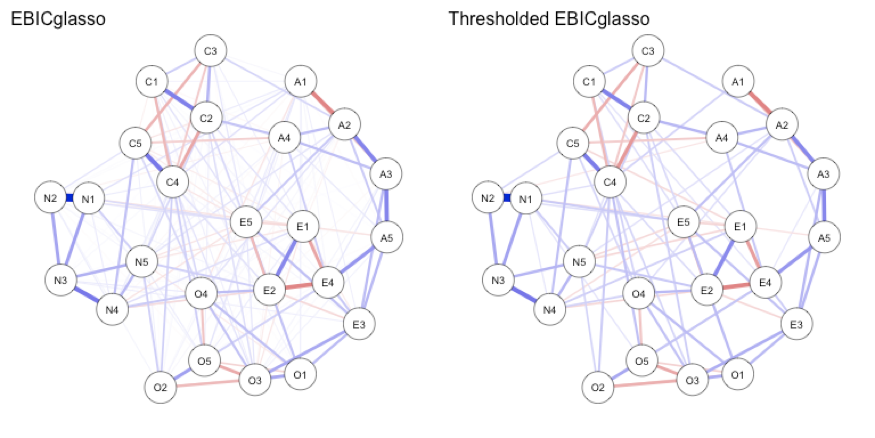While the thresholded graph is much sparser, that does not mean all removed edges are false positives. Many are likely reflecting true edges. ## Unregularized Model Search While the LASSO has mostly been studied in high-dimensional low-sample cases, in many situations research focuses on relatively low-dimensional (e.g., 20 nodes) settings with high sample size (e.g., $$N > 1{,}000$$). To this end, it is arguable if regularization techniques are really needed. In the particular case of GGMs, one could also use model selection on unregularized models in which some pre-defined edge-weights are set to zero. It has been shown that (extended) Bayesian information criterion (EBIC) selection of such unregularized models selects the true model as $$N$$ grows to $$\infty$$ (Foygel and Drton, 2010). The new function ggmModSelect now supports model search of unregularized GGM models, using the EBIC exactly as it is computed in the Lavaan package. The hypertuningparameter is set by default to $$0$$ (BIC selection) rather than $$0.5$$, as preliminary simulations indicate $$\gamma = 0$$ shows much better sensitivity while retaining high specificity. By default, ggmModSelect will first run the glasso algorithm for $$100$$ different tuning parameters to obtain $$100$$ different network structures. Next, the algorithm refits all those networks without regularization and picks the best. Subsequently, the algorithm adds and removes edges until EBIC can no longer be improved. The full algorithm is: 1. Run glasso to obtain 100 models 2. Refit all models without regularization 3. Choose the best according to EBIC 4. Test all possible models in which one edge is changed (added or removed) 5. If no edge can be added or changed to improve EBIC, stop here 6. Change the edge that best improved EBIC, now test all other edges that would have also lead to an increase in EBIC again 7. If no edge can be added or changed to improve EBIC, go to 4, else, go to 6. When stepwise = FALSE, steps 4 to 7 are ignored, and when considerPerStep = "all", all edges are considered at every step. The following codes showcase the algorithm: modSelect_0 <- ggmModSelect(cor_auto(bfiSub), nrow(bfi), gamma = 0, nCores = 8) modSelect_0.5 <- ggmModSelect(cor_auto(bfiSub), nrow(bfi), gamma = 0.5, nCores = 8)  layout(t(1:2)) g3 <- qgraph(modSelect_0$graph, layout = g1$layout, theme = "colorblind", title = "ggmModSelect (gamma = 0)", cut = 0) g4 <- qgraph(modSelect_0.5$graph, layout = g1\$layout, theme = "colorblind",
title = "ggmModSelect (gamma = 0.5)", cut = 0)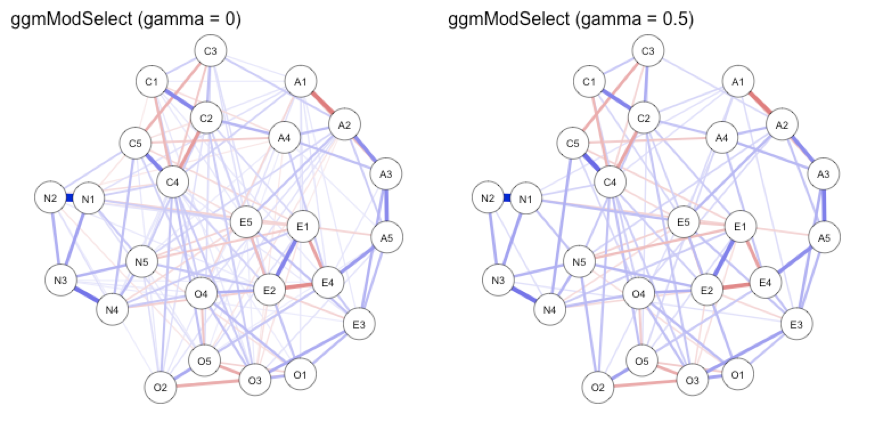Note that this algorithm is very slow in higher dimensions (e.g., above 30-40 nodes), in which case only the regular EBICglasso, thresholded EBICglasso, or setting stepwise = FALSE are feasible. Of note, centrality analyses, especially of the more stable strength metric, are hardly impacted by the estimation method:

centralityPlot(
list(EBICglasso=g1,
EBICglasso_threshold=g2,
ggmModSelect_0 = g3,
ggmModSelect_0.5 = g4
))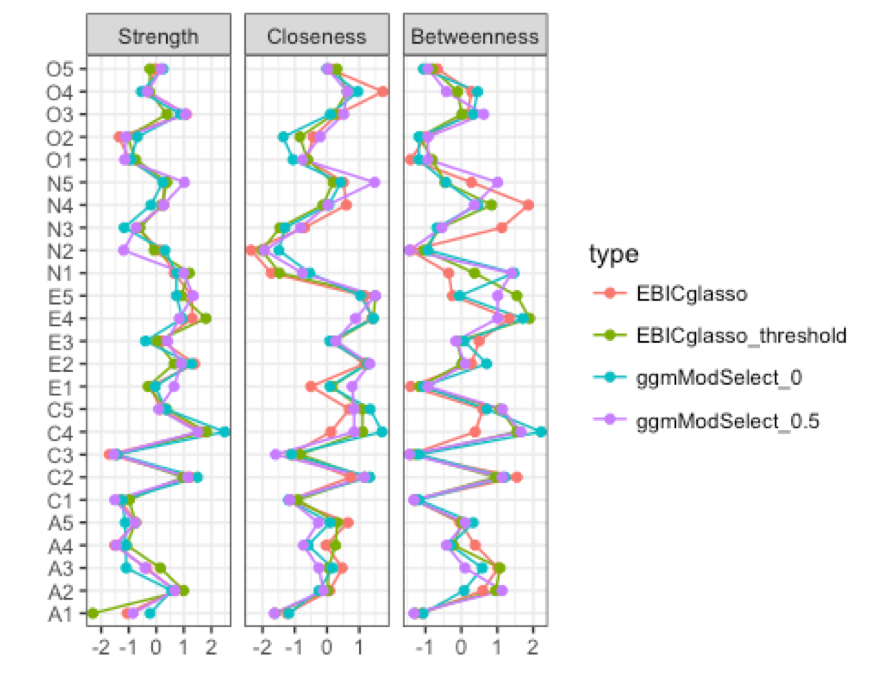Both thresholded EBICglasso and ggmModSelect are implemented in the development version of bootnet, which will be updated soon to CRAN as well. Preliminary simulations show that both guarantee high specificity, while losing sensitivity. Using ggmModSelect with $$\gamma = 0$$ (BIC selection) shows better sensitivity and works well in detecting small edges, but is slow when coupled with stepwise model search, which may make bootstrapping hard. I encourage researchers to investigate these and competing methods in large-scale simulation studies.

## Which estimation method to use?

Both new methods are much more conservative than the EBICglasso, leading to drops in sensitivity and possible misrepresentations of the true sparsity of the network structure. For exploratory hypothesis generation purposes in relatively low sample sizes, the original EBICglassso is likely to be preferred. In higher sample sizes and with a focus point on identifying small edges, the conservative methods may be preferred instead. There are many more GGM estimation procedures available in other R packages, and detailed simulation studies investigating which estimator works best in which case are now being performed in multiple labs. I have also implemented simulation functions in the developmental version of bootnet to aide studying these methods, which I will describe in an upcoming blog post.

# Flow diagrams

Sometimes, researchers are interested in the connectivity of one node in particular, which can be hard to see in the Fruchterman-Reingold algorithm, especially when the connections to that one node are weak. The new flow function, which I developed together with Adela Isvoranu, can be used to place nodes in such a way that connections of one node are clearly visible. The function will place the node of interest to the left, then in vertical levels nodes connected to the node of interest with 1, 2, 3, etcetera edges. Edges between nodes in the same level are displayed as curved edges. For example:

flow(g2, "N3", theme = "colorblind", vsize = 4)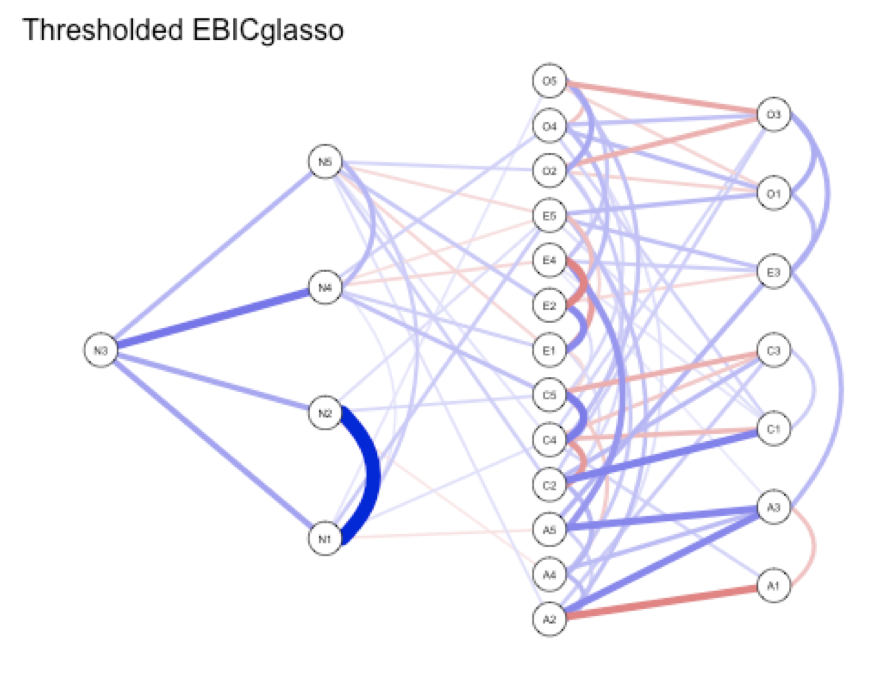# Expected influence

The centrality index expected influence is now returned by centrality() and can be plotted using centralityPlot(), although it has to be requested using include. In addition, the plots can now be ordered by one of the indices:

centralityPlot(g2, include = c("Strength","ExpectedInfluence"),
orderBy = "ExpectedInfluence")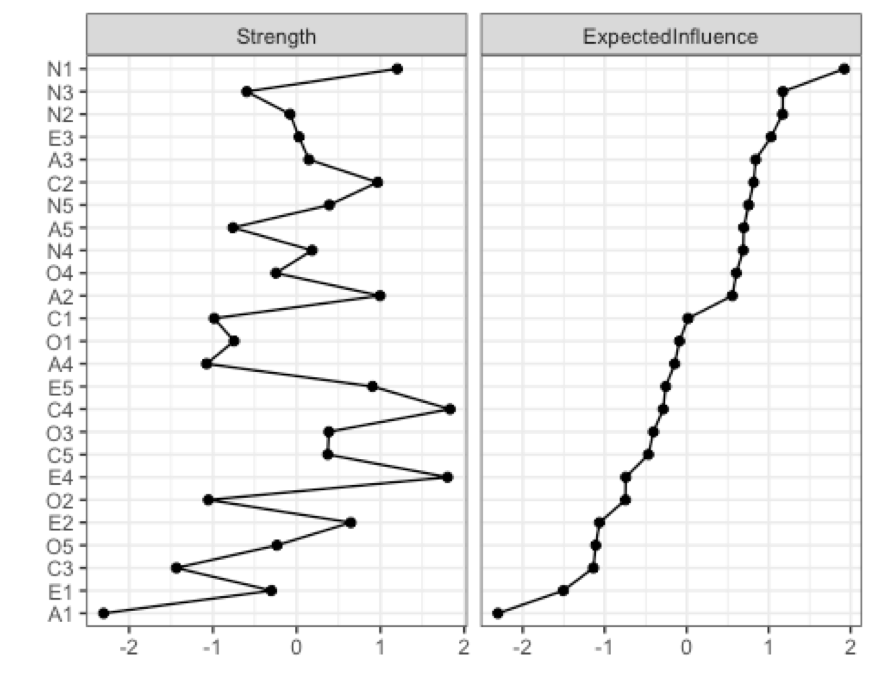Note, however, that the BFI network is not an optimal network to compute expected influence on, as some variables are (arbitrarily) scored negatively. It is best to compute expected influence on a network in which higher scores on all nodes have the same interpretation (e.g., symptoms in which higher = more severe).

# Future developments

As always, I highly welcome bug reports and code suggestions on Github. In addition, I will also update the bootnet package soon and write a separate blog post on its latest additions.

# References

Jankova, J., and van de Geer, S. (2018) Inference for high-dimensional graphical models. In: Handbook of graphical models (editors: Drton, M., Maathuis, M., Lauritzen, S., and Wainwright, M.). CRC Press: Boca Raton, Florida, USA.

Foygel, R., & Drton, M. (2010). Extended Bayesian information criteria for Gaussian graphical models. In Advances in neural information processing systems (pp. 604-612).